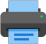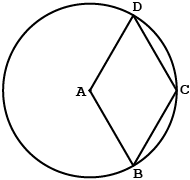Lista de exercícios do ensino médio para impressãoSelect all.
print selected exercises
The picture shows the rhombus ABCD and the point A is the center of the circle that has a 4 cm length radius. Find the area of the rhombus in square centimeters.
a)
$\,4\sqrt{3}\,$
b)
$\,8\,$
c)
$\,12\,$
d)
$\,8\sqrt{3}\,$
e)
$\,12\sqrt{3}\,$